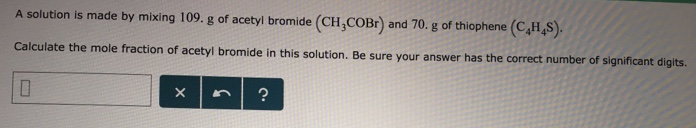# Problem: A solution is made by mixing 109. g of acetyl bromide (CH3COBr) and 70. g of thiophene (C4H4S). Calculate the mole fraction of acetyl bromide in this solution. Be sure your answer has the correct number of significant digits.

###### FREE Expert Solution
90% (172 ratings)###### Problem Details

A solution is made by mixing 109. g of acetyl bromide (CH3COBr) and 70. g of thiophene (C4H4S). Calculate the mole fraction of acetyl bromide in this solution. Be sure your answer has the correct number of significant digits.